Courses
Courses for Kids
Free study material
Free LIVE classes
More

# Number Sense - Fun Examples and ActivitiesLIVE
Join Vedantu’s FREE Mastercalss

## Introduction to Number Sense

So what is number sense? Number sense simply means the group of skills that will allow children to work with numbers. These skills are very important for performing mathematical operations. In this session, we will learn the basic concept of number skills and also a few solved examples.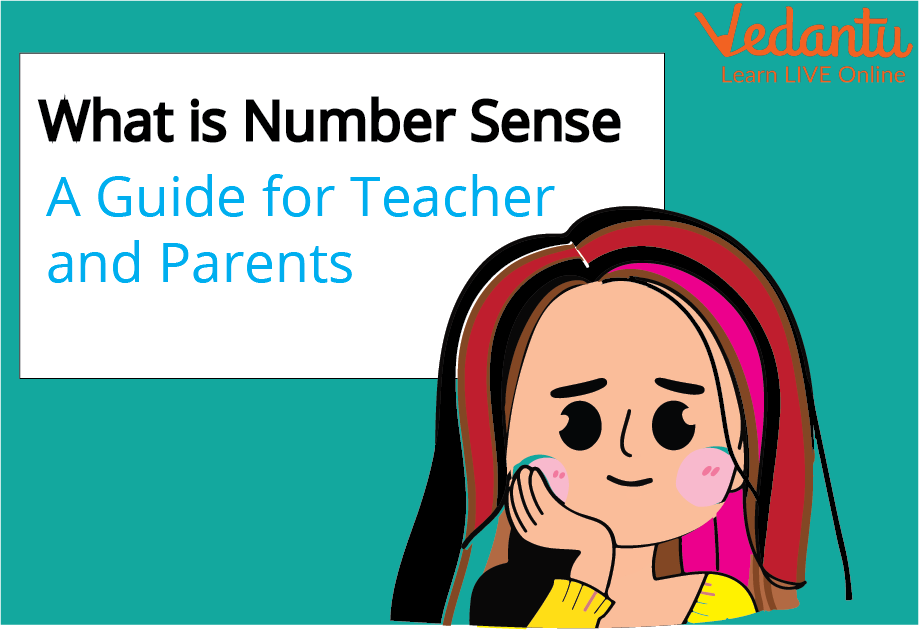### What is Number Sense?

Number sense could be easily defined as the ability of a child to understand, connect and relate the numbers with each other. Having a strong number sense is very important in children as it would allow the child to think flexibly and fluently about numbers. Number sense allows the child to create a healthy relationship with the numbers and to be able to talk and love maths as a language.

Number sense involves

• The ability to understand the quantities.

• Understanding the concept of bigger and smaller numbers. Children with good number sense will easily separate numbers which are less or big when asked.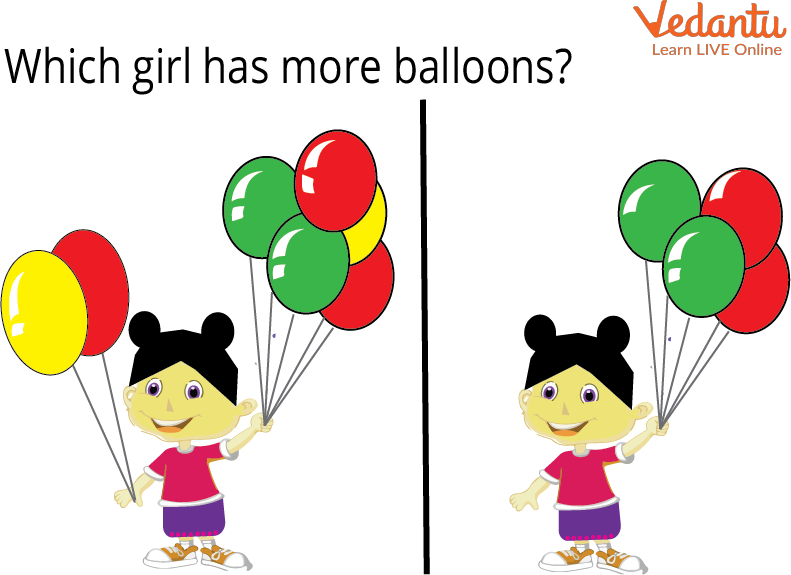• The ability to understand the order of numbers such as the 1st, 2nd, 4th etc.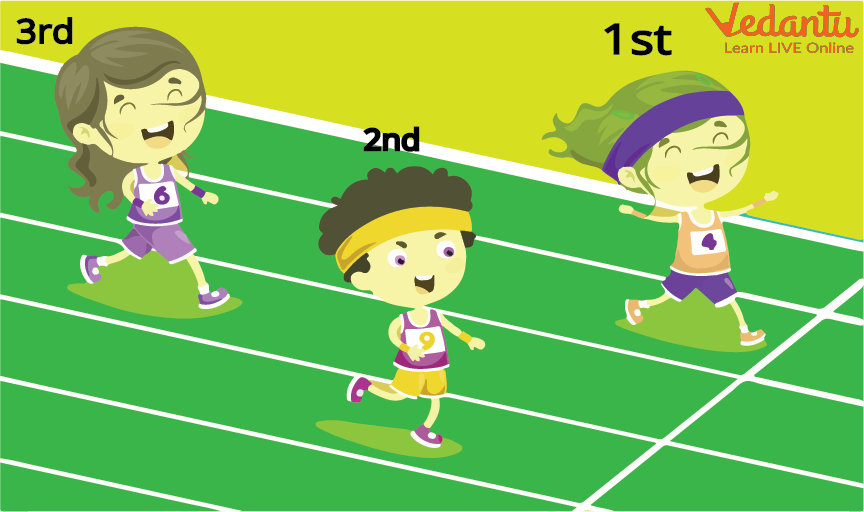• Having the ability to compare numbers and know which number is greater or smaller than the other number.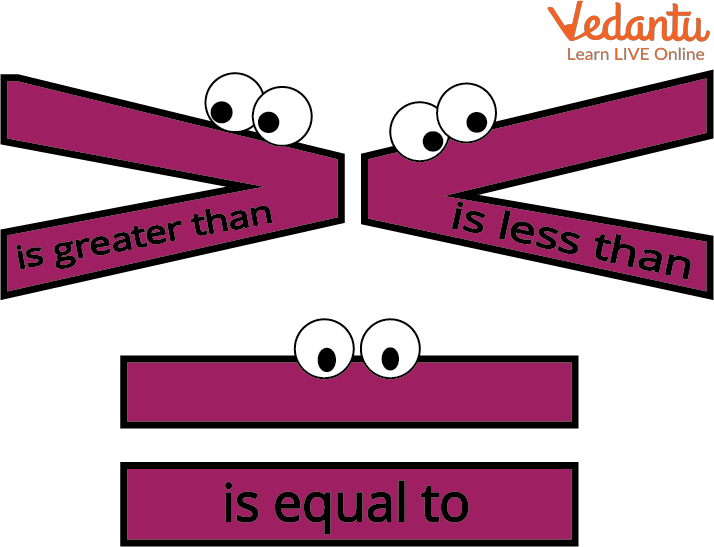• Having the ability to differentiate single-digit numbers and multiple digit numbers.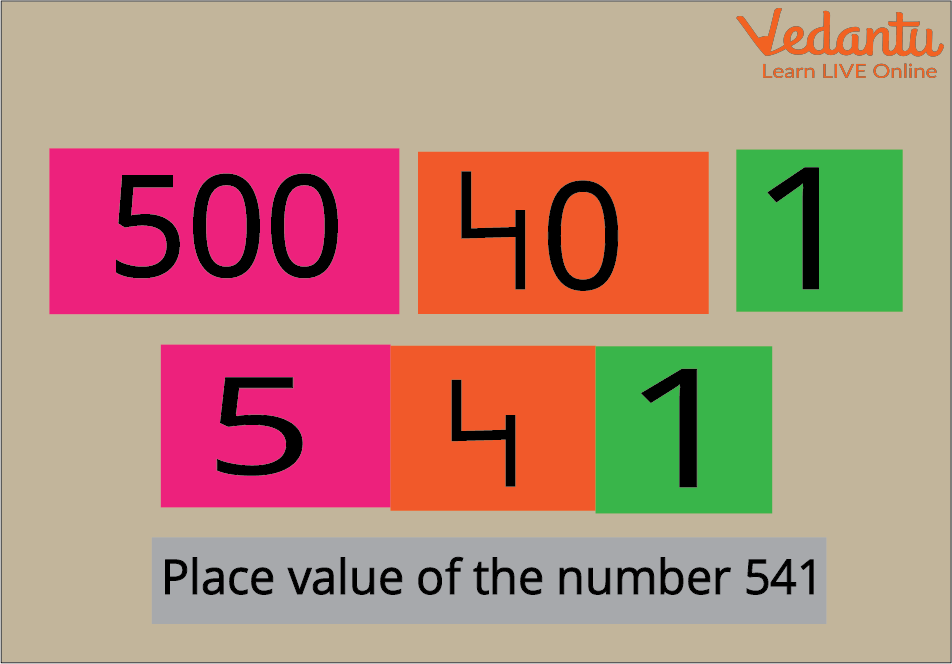### Advantages of having a strong Number Sense in Children.

Having a strong number sense will help the children to

• Talk comfortably and visualise the numbers. A child who has good number sense will be able to easily connect numbers and solve them.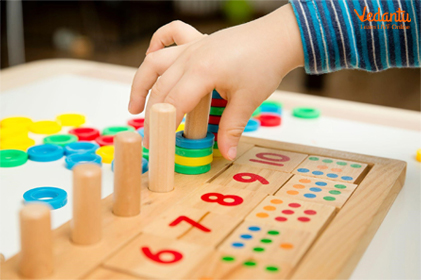• Solve the Problems Mentally - Having good number sense will  help in solving the problems in mind without the use of pen and paper.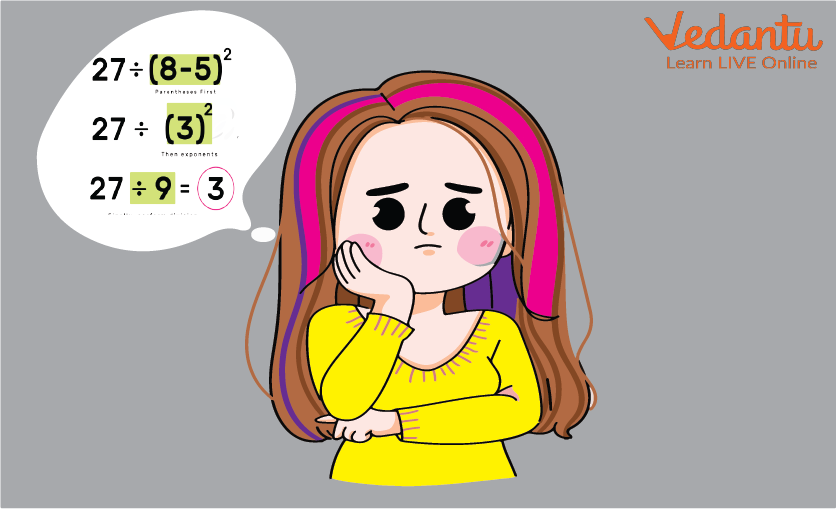• Separating the Numbers and Putting them back together in different Ways - Number sense examples, Suresh was asked to break down 10 rupees coin in different ways. He went on and easily said that a 10 rupees coin can be easily added as 5+ 5, 6+ 4, 8+ 2, 0+10 and so on. This will only help every child to learn all the ways to make 20.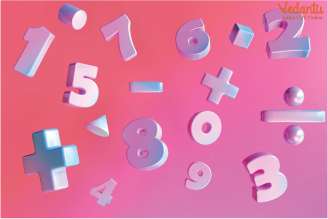### How to improve Number Sense in Children?

Here are a few ideas that will surely help in promoting number sense in children:

• Create events that bring maths into a child’s everyday life. For example, ask the child to count the number of steps it takes to reach home from the School Van or ask them to count the number of doors or windows in any building. This will help the children to include numbers in day to day life.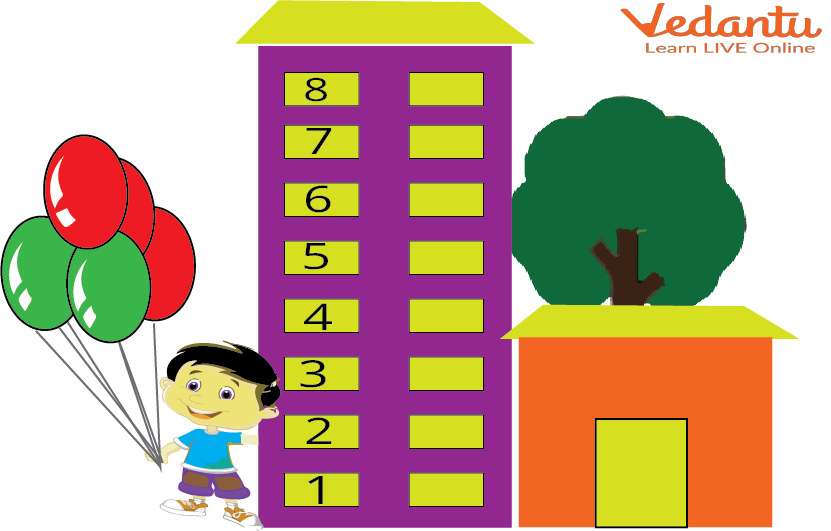• Allow them to think about maths with an open mind. For example Instead of asking them to tell you what is 10+5? ask them 'What are different ways to make 15? '. This method will surely allow more flexible thinking and allow the child to know more than one answer.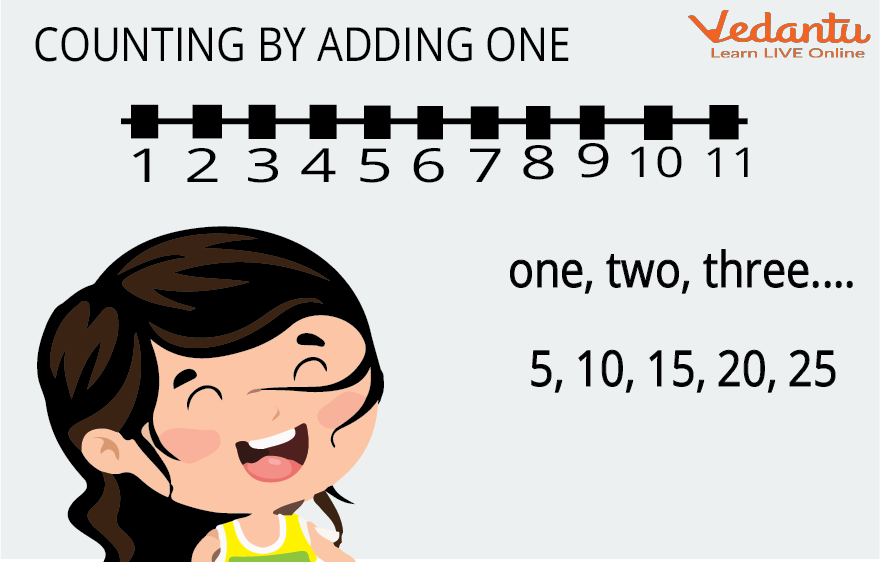• Boost their Confidence - Exercising is a great way to give children confidence so they won't fear doing complex problems in life. For children, give them small problems such as telling them to count the number of fingers in their hands• Help them Play with Numbers - This method literally means that children should be given toys that would help them count and separate the numbers when asked. For example, Legos could be used to count the object and write the numbers.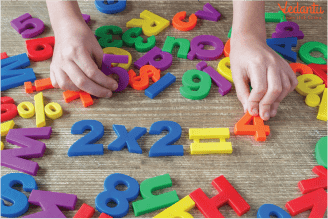### Solved Questions

Q1: What is the expanded form of the number 126?

Ans: It is given that the number is 126.

The following number can be written as : (1x100)+ (2x10) + (6x1)

1 is at the hundred’s position, two is present at the ten’s position, and six is present at the one’s position. If we place them in the one’s period we have.

One’s period

 Hundreds Ones 1 0 0 2 0 6

Hence the number in the expanded form is One hundred and twenty-six.

Q2.What is the predecessor of 1000

Ans: It is given that the number is 1000 and we have to find the predecessor. The predecessor is one less than the natural number. So we just have to subtract 1 from 1000 and thus the answer is 1000-1 = 999.

Q3. What is the successor of 1500

Ans: It is given that the number is 1500 and we have to find the successor. A successor is a term that would come after a particular number or term or a value. If the number is n then its successor would be n+1.

Hence the successor of 1500 is 1501.

### Fun Facts:

• The number 0 is also known as zero, nought, naught, nil, zilch, and zip.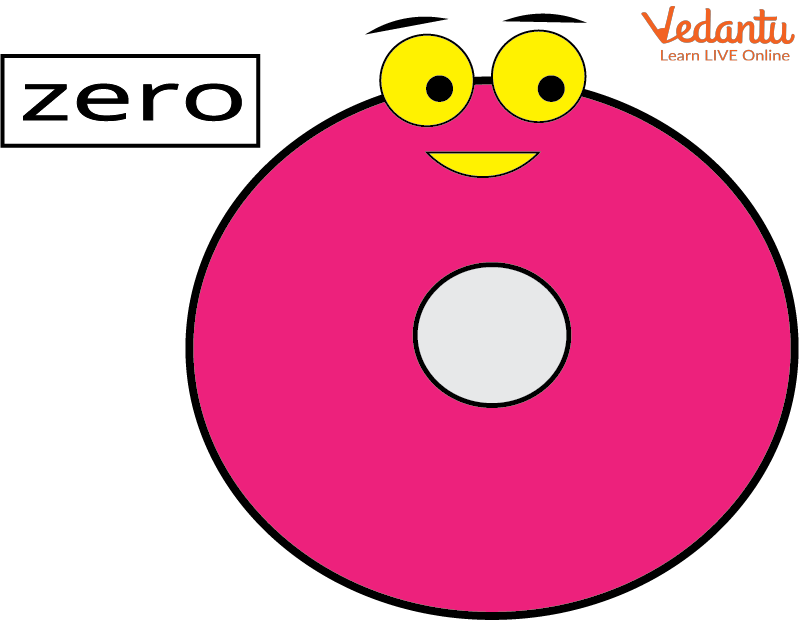• 2, 3, 5, 7, 11, 13, 17, 19, 23, and 29 are the ten smallest prime numbers.

• The only prime numbers that end in a 2 or a 5 are 2 and 5.

• The Hindu-Arabic numeral system, which was developed over 1000 years ago, is the basis for the numerical digits we use today, such as 1, 2, and 3.

### Summary

A set of important numerical skills is referred to as "number sense." Early emphasis on number sense lays the foundation for later grades when it comes to calculating and solving more complex problems. It involves the capability to understand numerical values as well as concepts like more and less.

### Learning By Doing

1. Radha’s parents have a different way of celebrating Diwali as she was born that day. They buy sweets double Radha’s weight and distribute them among the poor people.

When Radha was born she was just 3 kg. Today is Diwali and Radha is 5 years old and she is 28 kg now. Now with the help of you, parents guess the weight and the number of sweets her parents distribute every Diwali.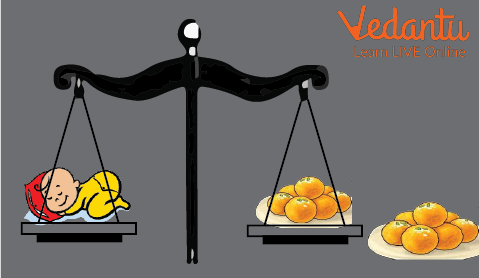The trick to solve: Radha’s parents buy and distribute sweets that double her weight every year, so you just have to know her weight of Radha every year and double it.

2. Make a list of  all the things which  are bought at home. Find out how much of each thing is bought at one time. These things could be anything from onions, tomatoes, sugar, salt, onions, milk etc.The trick to solve: Take the help of your parents to solve this easy task.

 Name of Things How much is bought
Last updated date: 23rd Sep 2023
Total views: 147.6k
Views today: 4.47k

## FAQs on Number Sense - Fun Examples and Activities

1. Who invented the number system?

The number system was created by Indians. Aryabhatta invented place-value notation in the fifth century. Brahmagupta invented the zero sign a century later.

2. What is the difference between number and numeral?

Numeral is a word that describes a number and a number is expressed in digits. For example, Six  is an example of a numeral and its digit representation is 6.

3. What are counting numbers?

Natural numbers that can be counted are known as counting numbers. They begin with number one and go on to numbers two, three, four, and so on. We can't count 0 so it's not included in counting numbers.